Function Repository Resource:

# StringPermutations

Get character permutations for a string

Contributed by: Arnoud Buzing
 ResourceFunction["StringPermutations"][string] generates a list of all possible permutations of the characters in string. ResourceFunction["StringPermutations"][string,n] gives all permutations containing at most n characters. ResourceFunction["StringPermutations"][string,{n}] gives all permutations containing exactly n characters.

## Details and Options

ResourceFunction["StringPermutations"] is implemented with Characters and Permutations.
ResourceFunction["StringPermutations"] is the String analog of Permutations of a List.

## Examples

### Basic Examples (3)

Permutations of "cat":

 In:=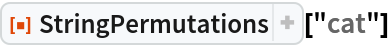Out=Three character permutations of "horse":

 In:=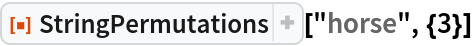Out=Zero, one, two and three character permutations of "garden":

 In:=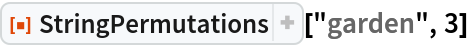Out=### Properties and Relations (1)

StringPermutations can also be implemented with the resource function StringFunction:

 In:=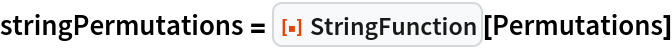Out=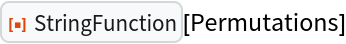In:=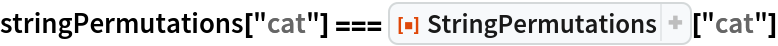Out=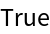In:=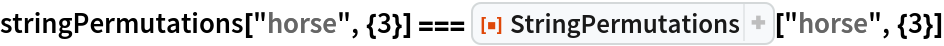Out=Arnoud Buzing

## Version History

• 1.0.0 – 01 June 2020